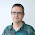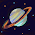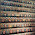## Saturday, May 26, 2012

### Creating a grid from scattered data using inverse of the distance with python (gdal_grid approach)

OK, I have to admit that I was so happy when I found the  scipy rbf function that I went too fast writing the entry about inverse of the distance.
I swear that the images are real (you can check it yourself), but when you change a few parameters or the point position, the result is really strange, definitely not the inverse of the distance algorithm I was thinking about.
Looking a little more around the gdal documentation, I found this explanation, which is very easy and clear:
http://www.gdal.org/grid_tutorial.html
The problem is that, at least in my python gdal version, is not possible to call the function directly, so I've been coding a little to get it.
All the example code and files can be downloaded in a zip file.

### First version: the algorithm

Putting all the code directly makes things difficult to understand, so first,  I'll reproduce the same program I wanted to show in the entrance, using matplotlib to show the results:

from math import pow
from math import sqrt
import numpy as np
import matplotlib.pyplot as plt

def pointValue(x,y,power,smoothing,xv,yv,values):
nominator=0
denominator=0
for i in range(0,len(values)):
dist = sqrt((x-xv[i])*(x-xv[i])+(y-yv[i])*(y-yv[i])+smoothing*smoothing);
#If the point is really close to one of the data points, return the data point value to avoid singularities
if(dist<0.0000000001):
return values[i]
nominator=nominator+(values[i]/pow(dist,power))
denominator=denominator+(1/pow(dist,power))
#Return NODATA if the denominator is zero
if denominator > 0:
value = nominator/denominator
else:
value = -9999
return value

def invDist(xv,yv,values,xsize=100,ysize=100,power=2,smoothing=0):
valuesGrid = np.zeros((ysize,xsize))
for x in range(0,xsize):
for y in range(0,ysize):
valuesGrid[y][x] = pointValue(x,y,power,smoothing,xv,yv,values)
return valuesGrid

if __name__ == "__main__":
power=1
smoothing=20

#Creating some data, with each coodinate and the values stored in separated lists
xv = [10,60,40,70,10,50,20,70,30,60]
yv = [10,20,30,30,40,50,60,70,80,90]
values = [1,2,2,3,4,6,7,7,8,10]

#Creating the output grid (100x100, in the example)
ti = np.linspace(0, 100, 100)
XI, YI = np.meshgrid(ti, ti)

#Creating the interpolation function and populating the output matrix value
ZI = invDist(xv,yv,values,100,100,power,smoothing)

# Plotting the result
n = plt.normalize(0.0, 100.0)
plt.subplot(1, 1, 1)
plt.pcolor(XI, YI, ZI)
plt.scatter(xv, yv, 100, values)
plt.title('Inv dist interpolation - power: ' + str(power) + ' smoothing: ' + str(smoothing))
plt.xlim(0, 100)
plt.ylim(0, 100)
plt.colorbar()

plt.show()


Let's explain it step by step:
1. The main method defines some sample data, calls the interpolation function and draws it using matplotlib. Just copy&paste, since it's long to explain.
2. invDist function: For each point in the coordinates matrix, a value needs to be calculated. First, a xSize*ySize zeroes values is calculated. Then for each point coordinate (the pixel position, in this simple case), a function that calculates the value is called.
3. This is the important part: We apply the formula: $V=\frac{\sum\limits_{i=1}^n \frac{v_{i}}{d_{i}^{p}}}{\sum\limits_{i=1}^n \frac{1}{d_{i}^{p}}}$ for each pixel.
• V is the interpolated value.
• n is the number of points
• d is the distance from the point to the pixel
• v is the value of the point
• p is the power we want to apply to the distance o weight it.
The distance is the Cartesian one, plus the smoothing factor (it gives more distance than the actual one, lowering the influence around the points). If the distance is close to the precision of the float numbers, we give the data value instead of the interpolated one, to avoid strange results.
And that's all. Here, the results (you can compare them with the ones in the other entry) which are consistent to the inverse of the distance method:
If the smoothing is zero and the power is high, the interpolation changes a lot around the points to give them their exact value.
If the smoothing is high and the power is one, the result is much smoother, but the values at the points are not maintained.

### Adding the GIS stuff:

The example above doesn't write to a GIS file. The function invDist must be changed so it creates the file using a gdal driver:
def invDist(xv,yv,values,geotransform,proj,xSize,ySize,power,smoothing,driverName,outFile):
#Transform geographic coordinates to pixels
for i in range(0,len(xv)):
xv[i] = (xv[i]-geotransform)/geotransform
for i in range(0,len(yv)):
yv[i] = (yv[i]-geotransform)/geotransform
#Creating the file
driver = gdal.GetDriverByName( driverName )
ds = driver.Create( outFile, xSize, ySize, 1, gdal.GDT_Float32)
if proj is not None:
ds.SetProjection(proj.ExportToWkt())
ds.SetGeoTransform(geotransform)
valuesGrid = np.zeros((ySize,xSize))
#Getting the interpolated values
for x in range(0,xSize):
for y in range(0,ySize):
valuesGrid[y][x] = pointValue(x,y,power,smoothing,xv,yv,values)

ds.GetRasterBand(1).WriteArray(valuesGrid)
ds = None
return valuesGrid

1. Note that the function will  need the geographic parameters geotransform and proj, as well as the output file name and driver.
2. First, the point positions must be changed to pixel locations in order to calculate distances
3. Then, the output file is created with the size and projection asked by the user
4. The values are calculated in the same way as in the other example
5. The file is written
The data in the first example was set into three lists, but the correct way to do it would be read it from a geometry file with the OGR library. Another function is defined to do that:

def readPoints(dataFile,Zfield='Z'):
data = {}
xv=[]
yv=[]
values=[]
ds = ogr.Open(dataFile)
if ds is None:
raise Exception('Could not open ' + dataFile)

layer = ds.GetLayer()
proj = layer.GetSpatialRef()
extent = layer.GetExtent()

feature = layer.GetNextFeature()
if feature.GetFieldIndex(zField) == -1:
raise Exception('zField is not valid: ' + zField)

while feature:
geometry = feature.GetGeometryRef()
xv.append(geometry.GetX())
yv.append(geometry.GetY())
values.append(feature.GetField(zField))

feature = layer.GetNextFeature()
data['extent'] = extent
data['xv']=xv
data['yv']=yv
data['values']=values
data['proj'] = proj
ds = None
return data


1. The function reads the point position and values from the given file and field name.
2. A dictionary is returned, to help retrieving all the values we may need to create the output raster. If no projection or geotransform is passed by the user, the input file extents and projection are used.
3. In the example files, a file called points.shp has some sample points to test the functions.
Finally, the main method must get the  parameters from the user, and call the other two methods to create the raster. I have copied some of the input options from the gdal_grid program, so it's easier to test the results. Of course, not all the options are implemented, but it shouldn't be difficult to do it.

if __name__ == "__main__":
#Setting the default values
power=2
smoothing=0

zField='Z'
dataFile=None
outFile=None
driverName='GTiff'
proj=None

geotransform = None
xMin=None
xMax=None
yMin=None
yMax=None
xSize=100
ySize=100

#Parsing the command line
argv = gdal.GeneralCmdLineProcessor( sys.argv )

i = 1
while i < len(argv):
arg = argv[i]
if arg == '-out_format':
driverName = argv[i+1]
driverName = driverName.replace("'","")
driverName = driverName.replace('"','')
i = i + 1
elif arg == '-zfield':
zField = argv[i+1]
zField = zField.replace("'","")
zField = zField.replace('"','')
i = i + 1
elif arg == '-a_srs':
proj = argv[i+1]
proj = proj.replace("'","")
proj = proj.replace('"','')
i = i + 1
elif arg == '-outsize':
xSize = argv[i+1]
xSize = xSize.replace("'","")
xSize = int(xSize.replace('"',''))
ySize = argv[i+2]
ySize = ySize.replace("'","")
ySize = int(ySize.replace('"',''))
i = i + 2
elif arg == '-txe':
xMin = argv[i+1]
xMin = xMin.replace("'","")
xMin = float(xMin.replace('"',''))
xMax = argv[i+2]
xMax = xMax.replace("'","")
xMax = float(xMax.replace('"',''))
i = i + 2
elif arg == '-tye':
yMin = argv[i+1]
yMin = yMin.replace("'","")
yMin = float(yMin.replace('"',''))
yMax = argv[i+2]
yMax = yMax.replace("'","")
yMax = float(yMax.replace('"',''))
i = i + 2
elif dataFile is None:
dataFile = arg
dataFile = dataFile.replace("'","")
dataFile = dataFile.replace('"','')
elif outFile is None:
outFile = arg
outFile = outFile.replace("'","")
outFile = outFile.replace('"','')
i = i + 1

if dataFile is None or outFile is None:
usage()
try:
data = readPoints(dataFile,zField)
except Exception,ex:
print ex
sys.exit(0)
if xMin is None:
xMin=data['extent']
xMax=data['extent']
if yMin is None:
yMin=data['extent']
yMax=data['extent']

geotransform=[]
geotransform.append(xMin)
geotransform.append((xMax-xMin)/xSize)
geotransform.append(0)
geotransform.append(yMax)
geotransform.append(0)
geotransform.append((yMin-yMax)/ySize)

if proj is None:
proj = data['proj']
else:
try:
proj = osr.SpatialReference()
proj.SetFromUserInput(str(proj))
except Exception,ex:
print ex
sys.exit(0)
#Creating the interpolation function and populating the output matrix value
try:
ZI = invDist(data['xv'],data['yv'],data['values'],geotransform,proj,xSize,ySize,power,smoothing,driverName,outFile)
except Exception,ex:
print ex
sys.exit(0)


1. First, the default parameter values are set. Some of them are None, since they are mandatory.
2. Then, the input arguments are read.
3. After having the input arguments, all the parameters that can be set, are set.
4. The function that reads the input file is run, and with it's result, the raster is created. Note that the geotransform is defined from the input file if no one is set by the user.
And the result is something like this when opened with QGis or any GIS:
The points are not coloured, but are the ones in the original file.
The whole code comes in the example file, with all the data you need to run the example.
To generate the picture above, the command is:
python invdistgis.py -zfield value points.shp out.tiff

### What's next

The original gdal_grid implements more interpolation methods (nearest value, and average). Doing the same in the script is as easy as adding other functions to use instead of pointValue.
Also, in the original program, an ellipse can be set to search only in some area around each pixel. This would be a little more difficult do implement, but is easy to find it in the gdal source code in a file called gdalgrid.cpp. I've put it in the example data.

As you can see, implementing this kind of stuff in python is not as difficult as it may seem at the beginning.

#### 23 comments:

1.This is excellent. Thanks! What are the units of xSize & ySize?

1.Thank you!
They are in pixel units, defining the final map size. Later, with the geotransform, the geographic position of each pixel is defined.

2.Ah, I see. After running the example, a single band tiff is produced that displays in QGIS as a solid gray image. Am I perhaps handling the image incorrectly? Thanks!

1.It's ok. You must right click the layer icon at the left panel, select properties, and configure the color scale you want. Depending on the QGIS version, this is done in different ways. In the example, I used "single band pseudocolor".

2.Perfect. Thank you Roger.

3.Sorry for all of the questions... I have a dataset in WGS83 coordinates which I would like to interpolate to a grid. Is it necessary to convert them to pixel units before interpolation, or may this method be modified to take points in such a coordinate system? I have found the following blog post that describes converting lon/lat to pixels but it seems there must be a more straightforward than converting to pixel then back to lat/lon: (http://monkut.webfactional.com/blog/archive/2012/5/2/understanding-raster-basic-gis-concepts-and-the-python-gdal-library/)

4.The program reads the projected data from a Shapefile (or any other format supported by OGR) and the output is in the same projection than the input data.

5.6.It is a great work. Thank you.
Is it possible get to the values of the grid points in ascii format. I was trying to interpolate 20 years precipitation station data to grid points. My station data are

rainfall.txt
station name lat (DD) long (DD) 01/01/14 01/02/14 01/03/14 01/04/14 01/05/14 01/06/14 01/07/14 01/08/14 01/09/14 01/10/14 01/11/14 01/12/14 01/13/14 01/14/14
A 1.5 1.4 A1 A2 A3 A4 A5 A6 A7 A8 A9 A10 A11 A12 A13 A14
B 2.1 3 B1 B2 B3 B4 B5 B6 B7 B8 B9 B10 B11 B12 B13 B14
C 2.3 0.7 C1 C2 C3 C4 C5 C6 C7 C8 C9 C10 C11 C12 C13 C14

my grid points are

grid.txt

Points lat long 01/01/14 01/02/14 01/03/14 01/04/14 01/05/14 01/06/14 01/07/14 01/08/14 01/09/14 01/10/14 01/11/14 01/12/14 01/13/14 01/14/14
1 1 1
2 1 2
3 1 3
4 1 4
5 2 1
6 2 2
7 2 3
8 2 4
9 3 1
10 3 2
11 3 3
12 3 4

I want to append the values of each day's interpolated data to the existing grid point.

Thanks.

7.Hi Abrham,

Well, the best solution in your case would be creating a csv file, and use it as the file with the points. How can you use a CSV file as an OGR input? I made a blog entry long time ago explaining it:
http://geoexamples.blogspot.com/2011/07/converting-excel-file-into-postgis.html

8.Thank you Roger,
I wrote a pyscript to interpolate from the point shape file. I face another problem when I am importing xy data. I have lots of missing data and the GIS understood as zero which in turn affects the interpolation. I am thinking of filling the missing station data before importing in the ArcGIS. If you have any suggestion, that would be very helpful.
Thanks

9.Hello Abraham,

I think that you will have to ignore this points in the script (assign them the value -9999, for instance, and then make the script not to use them). An other option is to remove the NODATA points before running the script.

By the way, if you want to usse the GDAL script, here you have how to do it (section Comma Separated Values): http://www.gdal.org/gdal_grid.html

10.Hi Roger,

Thank you very much. Really helpful. Is there anyway one could use this by specifying the power factor and using nearest neighbor instead of smoothing ?

Regards

Johan Ferguson

11.Hi Roger,

I have tried your code and works fine thanks!
However, when I tried putting my own xv, yv and values, I get just 3 circle pattern.

Only change = yv = [-13.917 , -13.85 , -13.915 , -13.815 , -13.5489, -13.553,-13.867 , -13.443,
-13.503 , -13.906 , -13.567 , -13.812]
xv = [-171.783 , -171.783 , -171.75 , -171.7806, -172.661 , -172.71,-171.75,-172.355,
-172.79 , -172.045 , -172.757 , -171.94]
values = [5275., 3334. , 3734.5 , 2922.7 ,\
2510. , 3517., 3413. , 3678.,\
2091. , 2910., 2920.8 , 9999.8]

12.I believe the invDist function is wrong but why has no-one else said so after so much time. The pointValue function needs the grid position not the grid index, so:
def invDist(xv,yv,values,xsize=100,ysize=100,power=2,smoothing=0):
valuesGrid = np.zeros((ysize,xsize))
for x in range(0,xsize):
for y in range(0,ysize):
valuesGrid[x][y] = pointValue(XI[x,y],YI[x,y],power,smoothing,xv,yv,values)
return valuesGrid

13.How do you do a kriging interpolation? Please help me﻿

1.You have several libraries that do this.
You can start here: https://github.com/bsmurphy/PyKrige

14.How to create Thiessen polygon using GDAL in python?

1.Hi Alem,
I'm sorry, I never tried it.

15.Hi Roger,
Thanks for this example! The one thing I'm struggling with is how to use this method with latitude/longitude coordinates as the x/y? Any advice would be appreciated!

1.The projection tou use is not important for the method, the results will be the same. Just think that using lat/lon, the distances over the Earth won't be the same in the lat and lon directions (unless working really close to the Equator), so the result may not be accurate.

2.Hello Roger,
Thank you for this nice post! It inspired me to look for a vectorized solution with numpy (see below).
Peter (www.HealthyNumerics.blog)

#---- input: create the input data as numpy arrays
xv = np.array([10,60,40,70,10,50,20,70,30,60])
yv = np.array([10,20,30,30,40,50,60,70,80,90])
values = np.array([1,2,2,3,4,6,7,7,8,10])

#---- run through the grid and get the interpolated value at each point
ZI = np.zeros_like(XI) # initialize with zero
for jx in range(nx):
for jy in range(ny):
ZI[jx,jy] = get_interpolatedValue(XI[jx,jy],YI[jx,jy])

#---- the vectorized interpolation without for-loops is now:
def get_interpolatedValue(xp,yp):
dx = xp - xv
dy = yp - yv
dd = np.sqrt(dx**2 + dy**2 + smoothing**2) # distance
dd = np.where(np.abs(dd) <10**(-3), 10**(-3), dd) # limit too small distances
dd = 1.0/dd**power # dd = weight = 1.0 / (dd**power)
dd = dd/np.sum(dd) # normalized weights
vi = np.dot(dd,values) # interpolated value = scalar product
return vi

16.unfortunately google-blogspot deletes the leading free spaces, but you'll find out how the code can run.
Peter